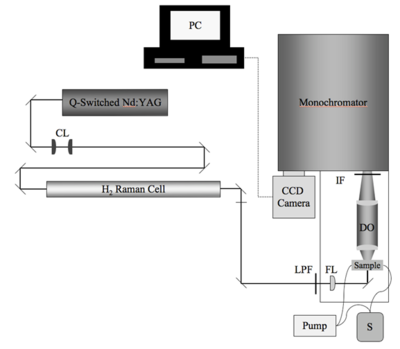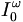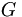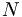# Hyper Rayleigh Scattering

Hyper Rayleigh Scattering (aka Harmonic Light Scattering or HRS) is one method for measuring the first hyperpolarizability β.

## Contents

### OverviewSchematic of the HRS setup. CL = collimating lenses; LPF = long-pass filter; FL = 300-mm focusing lens; S = solution of chromophore in solvent of choice; DO = detection optics; IF = interference filter (950 nm)

In HRS A dilute sample of a test chromophore is prepared in a solvent. An incident laser generates a second harmonic signal, specifically the frequency double signal from excitation of the sample molecules. This can be related to the beta of the sample using this formula:$I^ {2 \omega }_{HRS} = [ (I ^ \omega_0 \cdot T ^ \omega_{solv})^2 G ] ( N_{solv} \langle \beta ^ 2 _{solv} \rangle + N_{sam} \langle \beta ^ 2 _{sam} \rangle ) \,\!$

where$I ^ \omega_0\,\!$ is the intensity of the incident field$T ^ \omega_{solv}\,\!$ is the transmission of the fundamental radiation for the solvent$G\,\!$ is the instrumental efficiency$N\,\!$ is the number density of the sample (sam)and solvent (solv)$\beta^2\,\!$ is the orientational average of the square of the first hyperpolarizability.

See Clays 1992 . and Goovaerts 2001 

There are a number of ways the HRS characterization method is used in non-linear optics research.

The dielectric properties of the solvent influence the β (solvatochromatism). One area of research involves predicting and solvent effects so as to better understand the performance of molecules when densely packed in materials. Attempts have been made to calibrate HRS measurements with EFISH measurements of beta though they are based on different tensor elements.

See Wikipedia on Rayleigh Scattering

See Wikipedia on Raman Scattering

### Technique

The HRS measurement of β is a specialized device custom-built on an optics table. A series of mirrors, filters and devices hydrogen raman cell pass a specific wavelength of light into a sample which then emits light that is selected by a monochromator and then detected with a photocell. The components and configuration can change significantly from site to site. It is important to understand the significance of each portion of the setup. One possible HRS experimental setup is described here. (Press > to Play the video)